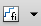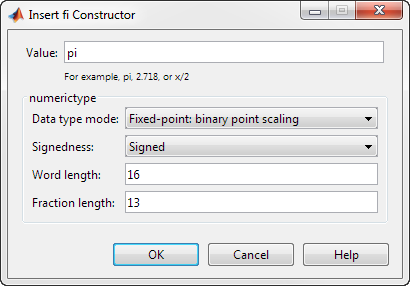Ways to Construct fi Objects

Types of fi Constructors

You can create fi objects using Fixed-Point Designer™ software in any of the following ways:

• You can use the fi constructor function to create a fi object.

• You can use the sfi constructor function to create a new signed fi object.

• You can use the ufi constructor function to create a new unsigned fi object.

• You can use any of the fi constructor functions to copy an existing fi object.

To get started, to create a fi object with the default data type and a value of 0:

a = fi(0)
a =

0

DataTypeMode: Fixed-point: binary point scaling
Signedness: Signed
WordLength: 16
FractionLength: 15

This constructor syntax creates a signed fi object with a value of 0, word length of 16 bits, and fraction length of 15 bits. Because you did not specify any fimath object properties in the fi constructor, the resulting fi object a has no local fimath.

To see all of the fi, sfi, and ufi constructor syntaxes, refer to the respective reference pages.

For information on the display format of fi objects, refer to View Fixed-Point Data.

Examples of Constructing fi Objects

The following examples show you several different ways to construct fi objects. For other, more basic examples of constructing fi objects, see the Examples section of the following constructor function reference pages:

Note

The fi constructor creates the fi object using a RoundingMethod of Nearest and an OverflowAction of Saturate. If you construct a fi from floating-point values, the default RoundingMethod and OverflowAction property settings are not used.

Constructing a fi Object with Property Name/Property Value Pairs

You can use property name/property value pairs to set fi and fimath object properties when you create the fi object:

a = fi(pi, 'RoundingMethod','Floor', 'OverflowAction','Wrap')
a =

3.1415

DataTypeMode: Fixed-point: binary point scaling
Signedness: Signed
WordLength: 16
FractionLength: 13

RoundingMethod: Floor
OverflowAction: Wrap
ProductMode: FullPrecision
SumMode: FullPrecision

You do not have to specify every fimath object property in the fi constructor. The fi object uses default values for all unspecified fimath object properties.

• If you specify at least one fimath object property in the fi constructor, the fi object has a local fimath object. The fi object uses default values for the remaining unspecified fimath object properties.

• If you do not specify any fimath object properties in the fi object constructor, the fi object uses default fimath values.

Constructing a fi Object Using a numerictype Object

You can use a numerictype object to define a fi object:

T = numerictype
T =

DataTypeMode: Fixed-point: binary point scaling
Signedness: Signed
WordLength: 16
FractionLength: 15
a = fi(pi, T)
a =

1.0000

DataTypeMode: Fixed-point: binary point scaling
Signedness: Signed
WordLength: 16
FractionLength: 15

You can also use a fimath object with a numerictype object to define a fi object:

F = fimath('RoundingMethod', 'Nearest',...
'OverflowAction', 'Saturate',...
'ProductMode','FullPrecision',...
'SumMode','FullPrecision')
F =

RoundingMethod: Nearest
OverflowAction: Saturate
ProductMode: FullPrecision
SumMode: FullPrecision
a = fi(pi, T, F)
a =

1.0000

DataTypeMode: Fixed-point: binary point scaling
Signedness: Signed
WordLength: 16
FractionLength: 15

RoundingMethod: Nearest
OverflowAction: Saturate
ProductMode: FullPrecision
SumMode: FullPrecision

Note

The syntax a = fi(pi,T,F) is equivalent to a = fi(pi,F,T). You can use both statements to define a fi object using a fimath object and a numerictype object.

Constructing a fi Object Using a fimath Object

You can create a fi object using a specific fimath object. When you do so, a local fimath object is assigned to the fi object you create. If you do not specify any numerictype object properties, the word length of the fi object defaults to 16 bits. The fraction length is determined by best precision scaling:

F = fimath('RoundingMethod', 'Nearest',...
'OverflowAction', 'Saturate',...
'ProductMode','FullPrecision',...
'SumMode','FullPrecision')
F =

RoundingMethod: Nearest
OverflowAction: Saturate
ProductMode: FullPrecision
SumMode: FullPrecision
F.OverflowAction = 'Wrap'
F =

RoundingMethod: Nearest
OverflowAction: Wrap
ProductMode: FullPrecision
SumMode: FullPrecision
a = fi(pi, F)
a =

3.1416

DataTypeMode: Fixed-point: binary point scaling
Signedness: Signed
WordLength: 16
FractionLength: 13

RoundingMethod: Nearest
OverflowAction: Wrap
ProductMode: FullPrecision
SumMode: FullPrecision

You can also create fi objects using a fimath object while specifying various numerictype properties at creation time:

b = fi(pi, 0, F)
b =

3.1416

DataTypeMode: Fixed-point: binary point scaling
Signedness: Unsigned
WordLength: 16
FractionLength: 14

RoundingMethod: Nearest
OverflowAction: Wrap
ProductMode: FullPrecision
SumMode: FullPrecision
c = fi(pi, 0, 8, F)
c =

3.1406

DataTypeMode: Fixed-point: binary point scaling
Signedness: Unsigned
WordLength: 8
FractionLength: 6

RoundingMethod: Nearest
OverflowAction: Wrap
ProductMode: FullPrecision
SumMode: FullPrecision
d = fi(pi, 0, 8, 6, F)
d =

3.1406

DataTypeMode: Fixed-point: binary point scaling
Signedness: Unsigned
WordLength: 8
FractionLength: 6

RoundingMethod: Nearest
OverflowAction: wrap
ProductMode: FullPrecision
SumMode: FullPrecision

Building fi Object Constructors in a GUI

When you are working with files in MATLAB®, you can build your fi object constructors using the Insert fi Constructor dialog box. After specifying the value and properties of the fi object in the dialog box, you can insert the prepopulated fi object constructor at a specific location in your file.

For example, to create a signed fi object with a value of pi, a word length of 16 bits and a fraction length of 13 bits:

1. On the Home tab, in the File section, click New > Script to open the MATLAB Editor.

2. On the Editor tab, in the Edit section, clickin the Insert button group. Click Insert fi... to open the Insert fi Constructor dialog box.

3. Use the edit boxes and drop-down menus to specify the following properties of the fi object:

• Value = pi

• Data type mode = Fixed-point: binary point scaling

• Signedness = Signed

• Word length = 16

• Fraction length = 134. To insert the fi object constructor in your file, place your cursor at the desired location in the file, and click on the Insert fi Constructor dialog box. Clicking closes the Insert fi Constructor dialog box and automatically populates the fi object constructor in your file:Determining Property Precedence

The value of a property is taken from the last time it is set. For example, create a numerictype object with a value of true for the Signed property and a fraction length of 14:

T = numerictype('Signed', true, 'FractionLength', 14)
T =

DataTypeMode: Fixed-point: binary point scaling
Signedness: Signed
WordLength: 16
FractionLength: 14

Now, create the following fi object in which you specify the numerictype property after the Signed property, so that the resulting fi object is signed:

a = fi(pi,'Signed',false,'numerictype',T)
a =

1.9999

DataTypeMode: Fixed-point: binary point scaling
Signedness: Signed
WordLength: 16
FractionLength: 14

Contrast the fi object in this code sample with the fi object in the following code sample. The numerictype property in the following code sample is specified before the Signed property, so the resulting fi object is unsigned:

b = fi(pi,'numerictype',T,'Signed',false)
b =

3.1416

DataTypeMode: Fixed-point: binary point scaling
Signedness: Unsigned
WordLength: 16
FractionLength: 14

Copying a fi Object

To copy a fi object, simply use assignment:

a = fi(pi)
a =

3.1416

DataTypeMode: Fixed-point: binary point scaling
Signedness: Signed
WordLength: 16
FractionLength: 13
b = a
b =

3.1416

DataTypeMode: Fixed-point: binary point scaling
Signedness: Signed
WordLength: 16
FractionLength: 13

Creating fi Objects For Use in a Types Table

You can write a reusable MATLAB algorithm by keeping the data types of the algorithmic variables in a separate types table. For example,

function T = mytypes(dt)
switch dt
case 'double'
T.b = double([]);
T.x = double([]);
T.y = double([]);

case 'fixed16'
T.b = fi([],1,16,15);
T.x = fi([],1,16,15);
T.y = fi([],1,16,14);
end
end

Cast the variables in the algorithm to the data types in the types table as described in Manual Fixed-Point Conversion Best Practices.

function [y,z]=myfilter(b,x,z,T)
y = zeros(size(x),'like',T.y);
for n=1:length(x)
z(:) = [x(n); z(1:end-1)];
y(n) = b * z;
end
end

In a separate test file, set up input data to feed into your algorithm, and specify the data types of the inputs.

% Test inputs
b = fir1(11,0.25);
t = linspace(0,10*pi,256)';
x = sin((pi/16)*t.^2);
% Linear chirp

% Cast inputs
T=mytypes('fixed16');
b=cast(b,'like',T.b);
x=cast(x,'like',T.x);
z=zeros(size(b'),'like',T.x);

% Run
[y,z] = myfilter(b,x,z,T);

Fixed-Point Designer DocumentationGet trial now